Nuclear Physics MSQ

# Nuclear Physics MSQ

Test Description

## 10 Questions MCQ Test Topic wise Tests for IIT JAM Physics | Nuclear Physics MSQ

Nuclear Physics MSQ for IIT JAM 2022 is part of Topic wise Tests for IIT JAM Physics preparation. The Nuclear Physics MSQ questions and answers have been prepared according to the IIT JAM exam syllabus.The Nuclear Physics MSQ MCQs are made for IIT JAM 2022 Exam. Find important definitions, questions, notes, meanings, examples, exercises, MCQs and online tests for Nuclear Physics MSQ below.
Solutions of Nuclear Physics MSQ questions in English are available as part of our Topic wise Tests for IIT JAM Physics for IIT JAM & Nuclear Physics MSQ solutions in Hindi for Topic wise Tests for IIT JAM Physics course. Download more important topics, notes, lectures and mock test series for IIT JAM Exam by signing up for free. Attempt Nuclear Physics MSQ | 10 questions in 45 minutes | Mock test for IIT JAM preparation | Free important questions MCQ to study Topic wise Tests for IIT JAM Physics for IIT JAM Exam | Download free PDF with solutions
 1 Crore+ students have signed up on EduRev. Have you?
*Multiple options can be correct
Nuclear Physics MSQ - Question 1

### For a radioactive substance with half life t1/2 and initial number of nuclei N0. The number the nuclei decayed in 3 half lives is :

Detailed Solution for Nuclear Physics MSQ - Question 1

Number of nuclei remaining after
n half lives is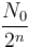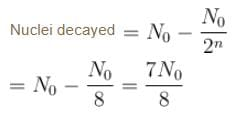The correct answer is: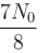*Multiple options can be correct
Nuclear Physics MSQ - Question 2

### A singly charged positive ion is accelerated through a potential difference of 1000V in a mass spectrograph. It then passes through a uniform magnetic field B = 1500 Gauss and then deflected into a circular path of radius 0.122 m.  Select the correct statements

Detailed Solution for Nuclear Physics MSQ - Question 2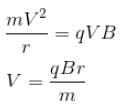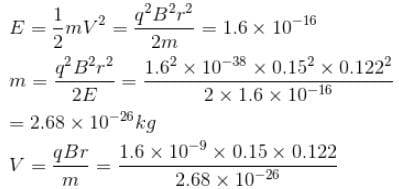= 1.09 × 105 m/s

The correct answers are: The speed of the ion is 1.09 × 105 m/s, The mass of the ion is 2.68 × 10–26 kg

*Multiple options can be correct
Nuclear Physics MSQ - Question 3

### A singly charged positive ion is accelerated through a potential difference of 1000V in a mass spectrograph. It then passes through a uniform magnetic field B = 1500 Gauss and then deflected into a circular path of radius 0.122 m. The mass number of the ion is :

Detailed Solution for Nuclear Physics MSQ - Question 3

Mass No. = No. of p + No. of neutron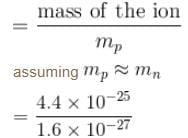= 2.75 × 102
A = 275
The correct answer is: 275

*Multiple options can be correct
Nuclear Physics MSQ - Question 4

Choose the correct option for mirror nuclei

Detailed Solution for Nuclear Physics MSQ - Question 4

Mirror nuclei have same A but the number of neutrons & protons are reversed.
There is a slight difference in the masses of a proton & neutron. This apart from the different coulomb force in mirror nuclei result in the difference in their masses.

The correct answers are: Nuclei with same mass number but the number of neutrons and protons reversed are called mirror nuclei, The mirror nuclei have different masses, The difference in masses is due to the difference in the proton & neutron masses & difference in the coulomb energy.

*Multiple options can be correct
Nuclear Physics MSQ - Question 5

1 mg of a radioactive material with half life 1600 years is kept for 2000 years. Choose the correct option.

Detailed Solution for Nuclear Physics MSQ - Question 5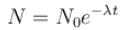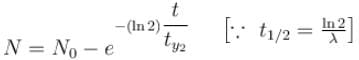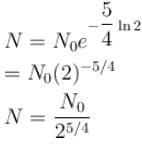mass remaining after 2000 years is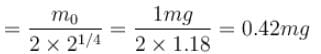mass decayed = 0.58 mg

The correct answers are: 0.42 mg  material was left after 2000 years, 0.58 mg  material decayed in 2000 years

*Multiple options can be correct
Nuclear Physics MSQ - Question 6

What are the reasons leading to the discovery of a neutrino in the case of a Beta decay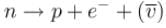Detailed Solution for Nuclear Physics MSQ - Question 6

The correct answers are: Continuous βray spectrum instead of a discrete spectrum as would have been the case had there only been an electron emitted, Most electrons were emitted with energy much less than maximum kinetic energy., Non conservation of angular momentum, Non conservation of linear momentum

*Multiple options can be correct
Nuclear Physics MSQ - Question 7

Nuclear force is

Detailed Solution for Nuclear Physics MSQ - Question 7

The correct answers are: spin dependent, charge independent, has repulsive core, same between two proton and two neutron

*Multiple options can be correct
Nuclear Physics MSQ - Question 8

From the Binding energy per nucleon curve, one can deduce that

Detailed Solution for Nuclear Physics MSQ - Question 8

B.E./Neutron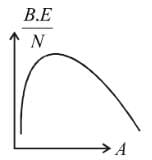Nuclei with smaller A fuse to form a nucleus of higher A which has more B.E./nucleon compared to the reactants. Nuclei with higher A(~200) fission into nuclei of smaller A which have higher B.E./nuclei  (thus they are more stable).

The correct answers are: Nuclei with lower mass number tend to undergo fusion, Nuclei with larger mass number tend to undergo nuclear fission

*Multiple options can be correct
Nuclear Physics MSQ - Question 9

Which of the following is true about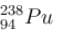Detailed Solution for Nuclear Physics MSQ - Question 9

Deuteron emission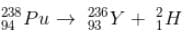α particle emission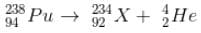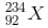has a lower mass number & from the B.E/nucleon v/s A plot, it can be concluded that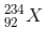will have a greater B.E./nucleon as compared to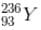∴  it would be more stable
∴  α decay is preferred

The correct answers are: 238Pu prefers to decay by emitting an α particle., Emitting an α particle results in a nucleus having greater binding energy per nucleon.

*Multiple options can be correct
Nuclear Physics MSQ - Question 10

Which of the following is true regarding the beam energies required to probe the inside of nucleus

Detailed Solution for Nuclear Physics MSQ - Question 10

For an electron beam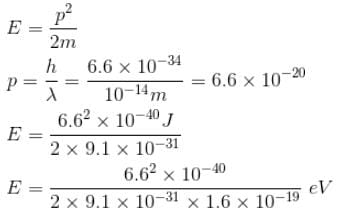~0.22 × 1010
= 2.2 × 109 eV
= 2.2 GeV
For proton beams,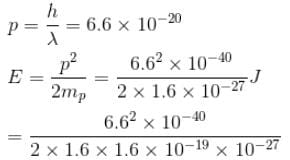= 8.5 × 106 eV

= 8.5 MeV

The correct answers are: The energy of a proton beam to probe the inside of a nucleus is of order MeV., The energy of an electron beam to probe the inside of a nucleus is of the order of GeV.

## Topic wise Tests for IIT JAM Physics

217 tests
 Use Code STAYHOME200 and get INR 200 additional OFF Use Coupon Code
Information about Nuclear Physics MSQ Page
In this test you can find the Exam questions for Nuclear Physics MSQ solved & explained in the simplest way possible. Besides giving Questions and answers for Nuclear Physics MSQ, EduRev gives you an ample number of Online tests for practice

217 tests